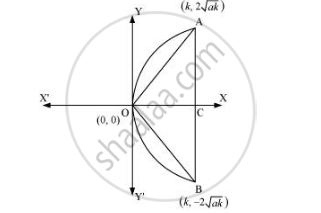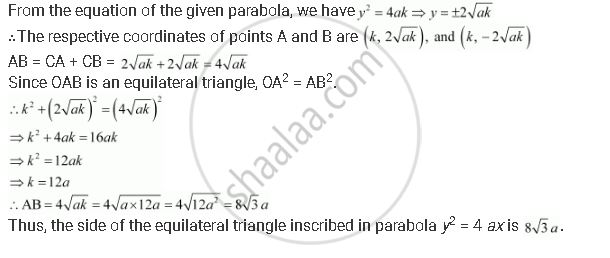CBSE (Arts) Class 11CBSE
Share
Notifications

View all notifications
Books Shortlist
Your shortlist is empty

# An Equilateral Triangle is Inscribed in the Parabola Y2 = 4 Ax, Where One Vertex is at the Vertex of the Parabola. Find the Length of the Side of the Triangle. - CBSE (Arts) Class 11 - Mathematics

Login
Create free account

Forgot password?
ConceptParabola Standard Equations of Parabola

#### Question

An equilateral triangle is inscribed in the parabola y2 = 4 ax, where one vertex is at the vertex of the parabola. Find the length of the side of the triangle.

#### Solution

Let OAB be the equilateral triangle inscribed in parabola y2 = 4ax.

Let AB intersect the x-axis at point C.Let OC = kIs there an error in this question or solution?

#### APPEARS IN

Solution An Equilateral Triangle is Inscribed in the Parabola Y2 = 4 Ax, Where One Vertex is at the Vertex of the Parabola. Find the Length of the Side of the Triangle. Concept: Parabola - Standard Equations of Parabola.
S